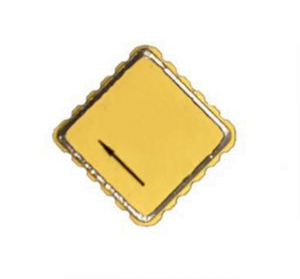Application

# What types of MEMS accelerometers are there?MEMS accelerometers can be divided into three forms according to the maturity of technology: piezoelectric, capacitive, and thermal.

1. The piezoelectric MEMS accelerometer uses the piezoelectric effect. There is a mass block supported by a rigid body inside. When there is movement, the mass block will generate pressure, the rigid body will generate strain, and the acceleration will be converted into an electrical signal output.
2. There is also a mass inside the capacitive sensing MEMS accelerometer. From the perspective of a single unit, it is a standard plate capacitor. The change of acceleration drives the movement of the active mass to change the distance between the two poles of the plate capacitor and the facing area, and the acceleration is calculated by measuring the capacitance change.
3. There is no mass block inside the thermal MEMS accelerometer. There is a heating body in the center, a temperature sensor around it, and a closed air cavity inside. Under the action of the heating body, the gas forms a hot air mass inside, the specific gravity of the hot air mass is different from the surrounding cold air. The thermal field change formed by the movement of the inertial hot air mass makes the sensor sense the acceleration value.Due to the existence of rigid body support inside the piezoelectric MEMS accelerometer, under normal circumstances, the piezoelectric MEMS accelerometer can only sense “dynamic” acceleration, but cannot sense “static” acceleration, which is what we call gravitational acceleration.  The capacitive and thermal types can sense both “dynamic” acceleration and “static” acceleration. The MEMS  Accelerometer used by ERICCO is capacitive.